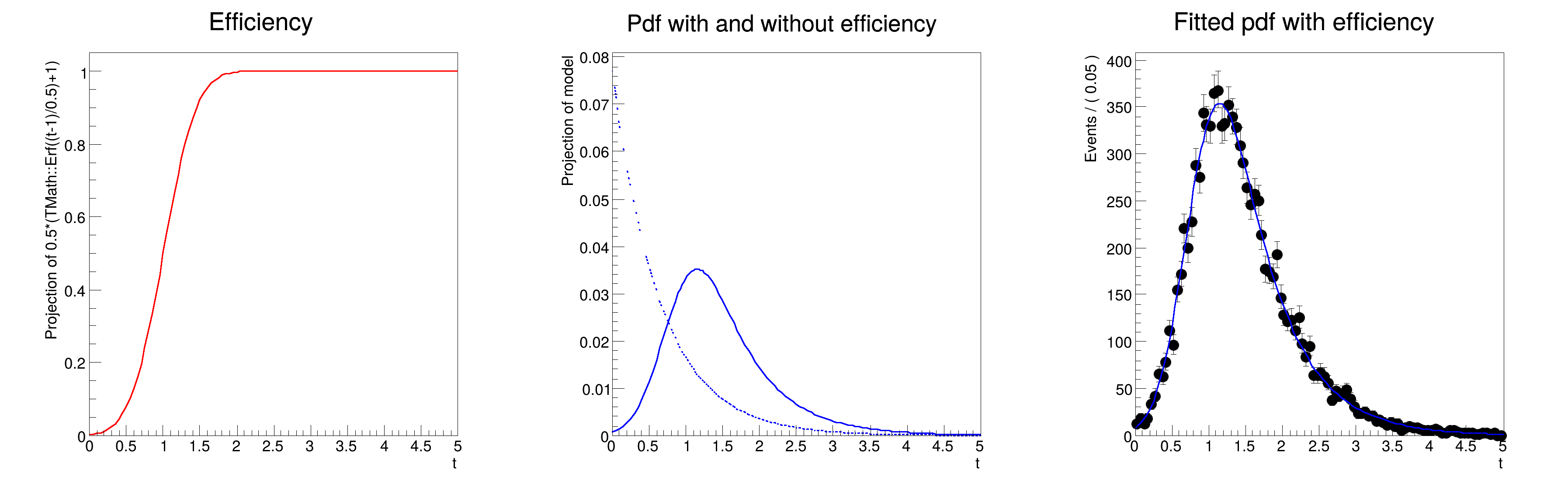ROOT   Reference Guide
Searching...
No Matches
rf703_effpdfprod.C File Reference

## Detailed DescriptionSpecial pdf's: using a product of an (acceptance) efficiency and a pdf as pdf#include "RooRealVar.h"
#include "RooDataSet.h"
#include "RooGaussian.h"
#include "RooConstVar.h"
#include "RooExponential.h"
#include "RooEffProd.h"
#include "RooFormulaVar.h"
#include "TCanvas.h"
#include "TAxis.h"
#include "RooPlot.h"
using namespace RooFit;
{
// D e f i n e o b s e r v a b l e s a n d d e c a y p d f
// ---------------------------------------------------------------
// Declare observables
RooRealVar t("t", "t", 0, 5);
// Make pdf
RooRealVar tau("tau", "tau", -1.54, -4, -0.1);
RooExponential model("model", "model", t, tau);
// D e f i n e e f f i c i e n c y f u n c t i o n
// ---------------------------------------------------
// Use error function to simulate turn-on slope
RooFormulaVar eff("eff", "0.5*(TMath::Erf((t-1)/0.5)+1)", t);
// D e f i n e d e c a y p d f w i t h e f f i c i e n c y
// ---------------------------------------------------------------
// Multiply pdf(t) with efficiency in t
RooEffProd modelEff("modelEff", "model with efficiency", model, eff);
// P l o t e f f i c i e n c y , p d f
// ----------------------------------------
RooPlot *frame1 = t.frame(Title("Efficiency"));
eff.plotOn(frame1, LineColor(kRed));
RooPlot *frame2 = t.frame(Title("Pdf with and without efficiency"));
model.plotOn(frame2, LineStyle(kDashed));
modelEff.plotOn(frame2);
// G e n e r a t e t o y d a t a , f i t m o d e l E f f t o d a t a
// ------------------------------------------------------------------------------
// Generate events. If the input pdf has an internal generator, the internal generator
// is used and an accept/reject sampling on the efficiency is applied.
std::unique_ptr<RooDataSet> data{modelEff.generate(t, 10000)};
// Fit pdf. The normalization integral is calculated numerically.
modelEff.fitTo(*data, PrintLevel(-1));
// Plot generated data and overlay fitted pdf
RooPlot *frame3 = t.frame(Title("Fitted pdf with efficiency"));
data->plotOn(frame3);
modelEff.plotOn(frame3);
TCanvas *c = new TCanvas("rf703_effpdfprod", "rf703_effpdfprod", 1200, 400);
c->Divide(3);
c->cd(1);
frame1->GetYaxis()->SetTitleOffset(1.4);
frame1->Draw();
c->cd(2);
frame2->GetYaxis()->SetTitleOffset(1.6);
frame2->Draw();
c->cd(3);
frame3->GetYaxis()->SetTitleOffset(1.6);
frame3->Draw();
}
#define c(i)
Definition RSha256.hxx:101
@ kRed
Definition Rtypes.h:66
@ kDashed
Definition TAttLine.h:48
Option_t Option_t TPoint TPoint const char GetTextMagnitude GetFillStyle GetLineColor GetLineWidth GetMarkerStyle GetTextAlign GetTextColor GetTextSize void data
The class RooEffProd implements the product of a PDF with an efficiency function.
Definition RooEffProd.h:19
Exponential PDF.
A RooFormulaVar is a generic implementation of a real-valued object, which takes a RooArgList of serv...
A RooPlot is a plot frame and a container for graphics objects within that frame.
Definition RooPlot.h:43
static RooPlot * frame(const RooAbsRealLValue &var, double xmin, double xmax, Int_t nBins)
Create a new frame for a given variable in x.
Definition RooPlot.cxx:239
TAxis * GetYaxis() const
Definition RooPlot.cxx:1279
void Draw(Option_t *options=nullptr) override
Draw this plot and all of the elements it contains.
Definition RooPlot.cxx:652
RooRealVar represents a variable that can be changed from the outside.
Definition RooRealVar.h:37
virtual void SetTitleOffset(Float_t offset=1)
Set distance between the axis and the axis title.
Definition TAttAxis.cxx:298
The Canvas class.
Definition TCanvas.h:23
The namespace RooFit contains mostly switches that change the behaviour of functions of PDFs (or othe...
[#1] INFO:NumericIntegration -- RooRealIntegral::init(modelEff_Int[t]) using numeric integrator RooRombergIntegrator to calculate Int(t)
[#1] INFO:NumericIntegration -- RooRealIntegral::init(modelEff_Int[t]) using numeric integrator RooRombergIntegrator to calculate Int(t)
[#1] INFO:Minimization -- RooAbsMinimizerFcn::setOptimizeConst: activating const optimization
[#1] INFO:Minimization -- The following expressions have been identified as constant and will be precalculated and cached: (eff)
[#1] INFO:Minimization -- RooAbsMinimizerFcn::setOptimizeConst: deactivating const optimization
[#1] INFO:NumericIntegration -- RooRealIntegral::init(modelEff_Int[t]) using numeric integrator RooRombergIntegrator to calculate Int(t)
Date
July 2008

Definition in file rf703_effpdfprod.C.